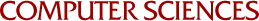# Dae Keun, Kim

Computer Sciences & Mathematics Undergraduate

 Resume Some geometry proofs 1 Some geometry proofs 2 Interests - Functional Languages such as Scheme, ML, and Prolog - Group Theory and Number Theory Professional Experience 2001-2004 Assistant Manager & Researcher, Technology & Information Dept. Nara-I-Net Co., Ltd., Seoul, Korea Computer Sciences Courses CS 240 - Introduction to Discrete Mathematics CS 302 - Introduction to Programming CS 352 - Digital System Fundamentals CS 354 - Machine Organization and Programming CS 367 - Introduction to Data Structures CS 412 - Introduction to Numerical Methods CS 520 - Introduction to Theory of Computation CS 536 - Introduction to Programming Languages and Compilers CS 537 - Introduction to Operating Systems CS 538 - Introduction to Theory and Design of Programming Languages CS 564 - Database Management Systems CS 577 - Introduction to Algorithms Mathematics Courses Math 221 - Calculus and Analytic Geometry I Math 222 - Calculus and Analytic Geometry II Math 234 - Calculus : Functions of Several Variables Math 331 - An Introduction to Probability and Markov Chain Models Math 340 - Elementary Matrix and Linear Algebra Math 371 - Basic Concepts of Mathematics Math 461 - College Geometry I Math 514 - Numerical Analysis Math 521 - Advanced Calculus Math 541 - Modern Algebra Math 567 - Elementary Number TheoryComputer Sciences | UW Home
 Feedback or content questions: send email to "dae" at the cs.wisc.edu server Technical or accessibility issues: lab@cs.wisc.edu Copyright © 2002, 2003 The Board of Regents of the University of Wisconsin System.